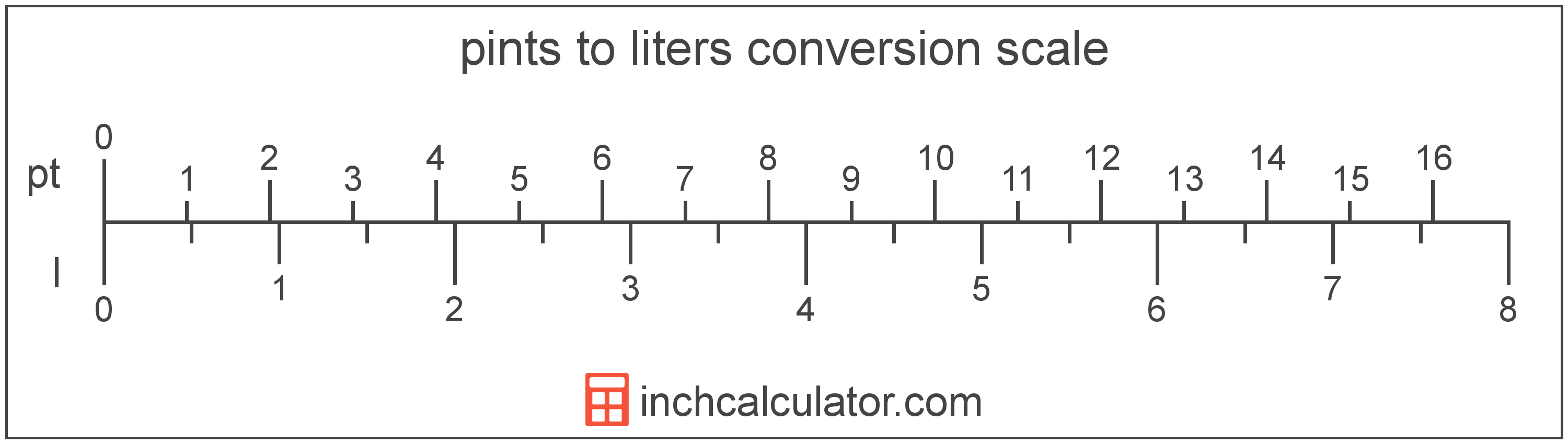# Liters to Pints Conversion

Enter the volume in liters below to get the value converted to pints.

Results in Pints:1 l = 2.113376 pt
Do you want to convert pints to liters?

## How to Convert Liters to PintsTo convert a liter measurement to a pint measurement, multiply the volume by the conversion ratio.

Since one liter is equal to 2.113376 pints, you can use this simple formula to convert:

pints = liters × 2.113376

The volume in pints is equal to the liters multiplied by 2.113376.

For example, here's how to convert 5 liters to pints using the formula above.
5 l = (5 × 2.113376) = 10.566882 pt

## Liters

A liter is a unit of volume equal to 1,000 cubic centimeters. The liter is a special name defined for the cubic decimeter and is exactly equal to the volume of one cubic decimeter.

The liter is an SI accepted unit for volume for use with the metric system. A liter is sometimes also referred to as a litre. Liters can be abbreviated as l, and are also sometimes abbreviated as L or . For example, 1 liter can be written as 1 l, 1 L, or 1 ℓ.

## Pints

The US liquid pint is a unit of fluid volume equal to one-eighth of a gallon, one-half of a quart, or two cups. The liquid pint should not be confused with the dry pint (US) or the imperial pint, which are different units.

The pint is a US customary unit of volume. Pints can be abbreviated as pt; for example, 1 pint can be written as 1 pt.

## Liter to Pint Conversion Table

Liter measurements converted to pints
Liters Pints
1 l 2.1134 pt
2 l 4.2268 pt
3 l 6.3401 pt
4 l 8.4535 pt
5 l 10.57 pt
6 l 12.68 pt
7 l 14.79 pt
8 l 16.91 pt
9 l 19.02 pt
10 l 21.13 pt
11 l 23.25 pt
12 l 25.36 pt
13 l 27.47 pt
14 l 29.59 pt
15 l 31.7 pt
16 l 33.81 pt
17 l 35.93 pt
18 l 38.04 pt
19 l 40.15 pt
20 l 42.27 pt
21 l 44.38 pt
22 l 46.49 pt
23 l 48.61 pt
24 l 50.72 pt
25 l 52.83 pt
26 l 54.95 pt
27 l 57.06 pt
28 l 59.17 pt
29 l 61.29 pt
30 l 63.4 pt
31 l 65.51 pt
32 l 67.63 pt
33 l 69.74 pt
34 l 71.85 pt
35 l 73.97 pt
36 l 76.08 pt
37 l 78.19 pt
38 l 80.31 pt
39 l 82.42 pt
40 l 84.54 pt

## References

1. National Institute of Standards and Technology, Units outside the SI, https://physics.nist.gov/cuu/Units/outside.html